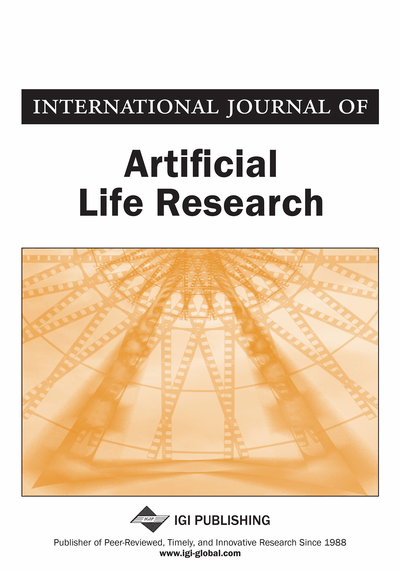# A Note on the Uniqueness of Positive Solutions for Singular Boundary Value Problems

Fu-Hsiang Wong, Sheng-Ping Wang, Hsiang-Feng Hong
DOI: 10.4018/ijalr.2011070105
OnDemand:
(Individual Articles)
Available
\$37.50
No Current Special Offers

## Abstract

In this paper, the authors examine sufficient condition for the uniqueness of positive solutions of singular Strum-Liouville boundary value problems. The authors use the uniqueness theorems of (E) with respect to the boundary conditions to show that the boundary value problems have one positive solution.
Article Preview
Top

## Introduction

In this paper, we focus on the uniqueness of positive solutions of boundary value problems for the nonlinear differential equation

(E) (ψ(u')u')' + f (t, u, u') = 0, θ1 < t < θ2,

subject to one of the following sets of boundary conditions:

where1,θ2) ⊆ (-∞,∞), ψC1(R;[0,∞)) with ψ'(y)y ≥ 0 on [0,∞) and f : (θ1,θ2)x(0,∞)x(-∞,∞)→(0,∞)

satisfies

(H1) f (t,x,y) is locally Lipschitz continuous for

(H2) f (t,x,y) / x is strictly decreasing with respect to x ∈ (o,∞) for each fixed (t,y) ∈ (θ1θ2) x (-∞,∞),

(H3) sgn(y) f (t,x,y) is decreasing with respect to y ∈ (-∞,∞)for each fixed (t,x) ∈ (θ1, θ2) x (0,∞),

(H4) there is a positive constant η > 0 such that f (t,x,y) is strictly monotonic with respect to x ∈ (0, η) for each fixed (t,y) ∈ (θ1, θ2) x (-∞,∞).

Furthermore, we use the uniqueness theorems of (E) with respect to the boundary conditions (BC.i) (i=1,2,3) to show that (BVP) mentioned previously has at most one positive solution in C1([θ1,θ2)].

Equations of the type (E) arise in studies of radially symmetric solutions (that is, solutions u that depend only on the variable r = |x|) of the m-Laplacian equation,R0 < |x| < R1, xRN, N ≥ 2

A radially symmetric solution of the above equation satisfies the ordinary differential equationR0<r<R1. With the change of variables,(for m ≠ N or t = log r (for m=N), it can be reduced to an equation of the typeθ1 < t < θ2, m ≥ 3.

Conditions for the existence of solutions of equation (E) with respect to (BC-1)-(BC.3) were studied by many authors: see for instance, De Figueiredo, Lions, and Nussbaum (1982), Granas, Guenther, and Lee (1985), Kaper, Knapp, and Kwong (1991), and Lions (1982). The uniqueness problem concerning (E), for the case m=2, has been studied by many authors. For example, Gatica, Oliker, and Waltman (1989), Kwong (1990), Brezis and Oswald (1986) and the excellent book by Agarwal and Lakshmikantham(1993). We further point out that Naito (1995) considered the caseand established some excellent conditions for uniqueness by using the well-known generalized Prüfer transformation and comparison theorems. In this article, the author attempts to afford a concise approach to study the uniqueness of positive solutions of (E) with boundary conditions (BC.1)-(BC.3) and (BC).

## Complete Article List

Search this Journal:
Reset
Open Access Articles: Forthcoming
Volume 8: 2 Issues (2018)
Volume 7: 2 Issues (2017)
Volume 6: 2 Issues (2016)
Volume 5: 1 Issue (2015)
Volume 4: 1 Issue (2014)
Volume 3: 4 Issues (2012)
Volume 2: 4 Issues (2011)
Volume 1: 4 Issues (2010)
View Complete Journal Contents Listing